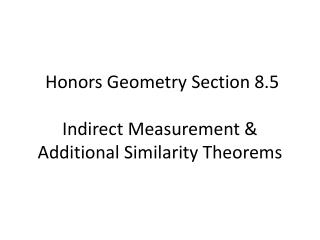# Honors Geometry Section 8.5 Indirect Measurement & Additional Similarity Theorems - PowerPoint PPT PresentationDownload PresentationHonors Geometry Section 8.5 Indirect Measurement & Additional Similarity Theorems

Honors Geometry Section 8.5 Indirect Measurement & Additional Similarity TheoremsDownload Presentation## Honors Geometry Section 8.5 Indirect Measurement & Additional Similarity Theorems

- - - - - - - - - - - - - - - - - - - - - - - - - - - E N D - - - - - - - - - - - - - - - - - - - - - - - - - - -
##### Presentation Transcript

1. Honors Geometry Section 8.5Indirect Measurement & Additional Similarity Theorems

2. Similar triangles can be used to find the height of objects.

3. Proportional Altitudes TheoremIf two triangles are similar, thencorresponding altitudes are proportional to a pair of corresponding sides.

4. Let’s think about why this theorem is true. Assume , why is ?

5. Proportional Medians Theorem If two triangles are similar, then corresponding medians are proportional to a pair of corresponding sides.

6. Proportional Angle Bisectors TheoremIf two triangles are similar, then corresponding angle bisectors are proportional to a pair of corresponding sides.

7. The triangles shown are similar. Solve for x.

8. The triangles shown are similar. Solve for x.

9. Proportional Segments TheoremAn angle bisector of a triangle divides the opposite side into segments which are proportional to the remaining two sides.

10. Solve for y.